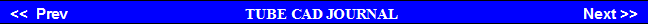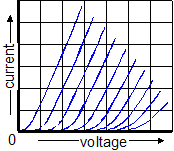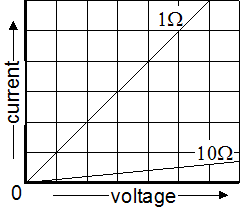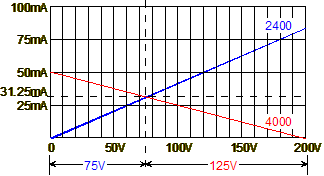We know we must start at the bottom left corner where voltage and current equals zero, as no voltage across a resistance means no current. The next point for the 1-ohm resistance would be one unit (1 volt) to the right and one unit up (1 amp); and for the 10-ohm resistance, it would be ten units to the right and one unit up. Remember, the lines continue infinitely, as the relationship between voltage and current defined by the line is constant.  So if we follow the 1-ohm line out to 100 kV, we know that the current will be 100 kA. Of course, no 1-ohm resistor on earth could withstand the test; but the issue here is pure resistances and not necessarily actual physical resistors.     The plotting of these two resistances reveals a key feature of graphing resistances: the lower the resistance, the steeper the line defined; and the higher the resistance, the flatter the line defined. This will prove valuable when working with tubes, as this principle allows quick inspection of plate resistances. Given that both graphs share the same X-Y scales, the steeper set of plate curves belongs to the vacuum tube with the lower plate resistance.     As the Ohm's Law formula is so simple, plotting different resistance values is usually of little value. But if we place two resistances in series and set a B+ voltage, then we can graphically see the voltage division between resistances.
 Extrapolation from Plate Curves      The detective has fingerprints; the fortune teller, a crystal ball; and the tube amplifier designer, plate curves. Each promises to reveal secrets. Who did it? What will the future bring? Will it work? Like the fingerprint, the tube's plate curves individualize the tube, which helps us decide which tube should be used. Like the crystal ball, a set of plate curves helps us predict how an amplifier will function, allowing us quickly to find the required grid voltage to set a given bias point and to determine maximum voltage swings. But before we tackle the vacuum tube's plate curves, we need to cover something much easier: simple resistances and straight lines.Simple Resistances   The movement from the graph's left to its right defines increasing voltage; from its bottom to its top, increasing current. This arrangement allows us graphically to display Ohm's Law:       Resistance = Voltage / Current. For example, 1 ohm equals 1 volt divided by 1 amp and 10 ohms equals 10 volts divided by 1 amp. Graphing these resistances is easy.
 Two Resistances In Series      When two resistances are in series (no resistance equaling zero) and are placed across a fixed voltage, one resistance will steal voltage from the other.www.tubecad.com   Copyright © 2003 GlassWare   All Rights

Pg.

6